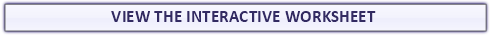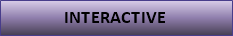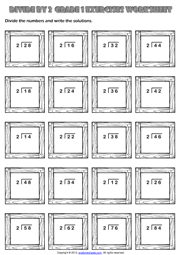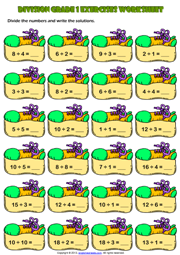# Grade 1 Division Printable Maths Worksheets and Exercises

Here on this page you can download and use Printable Grade 1 Maths Worksheets, Exercises, Handouts, Tests, Activities, Teaching and Learning Resources, Materials  for kids about Division

## First Grade Easy Division Exercise Maths Worksheet

A fun and easy division exercise maths worksheet for grade 1 (first grade) students and kids with trainers theme. Divide the numbers and write the solutions. (Horizontal Division Worksheet for kids single and double digits)

## Divide by 2 Maths Exercise Worksheet With Two Digits

An easy division exercise maths worksheet without remainders for grade 1 (first grade) students and kids with wooden frames theme. Divide the numbers by two and write the solutions. (Long Division Worksheet for kids)